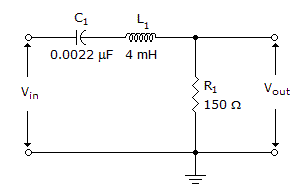# Electronics - RLC Circuits and Resonance - Discussion

### Discussion :: RLC Circuits and Resonance - General Questions (Q.No.26)

26.What is the bandwidth in the given circuit. If the winding resistance of the coil is 12?

 [A]. 6.4 kHz [B]. 47.2 kHz [C]. 53.7 kHz [D]. 60.2 kHz

Explanation:

No answer description available for this question.

 Deepti said: (May 18, 2011) R/2*3.141*L

 Kiran said: (Nov 2, 2011) fr=1/2*pi*sqrt(LC), q=1/r*sqrt(L/C), r=150+12=162; B.W= fr/q=6.4khz

 Monalisha said: (Dec 21, 2013) We know, q=wL/R OR 2*pi*f*L/R = f/B.W. f = 1/2*pi*sqrt(L*C) = 53650HZ = 53.65KHZ. q = 2*Pi*f*L/R = 8.32. B.W = f/q = 6.4KHZ.

 Emil Lagman said: (Mar 5, 2015) R = 150+12 = 162 ohms. BW = R/2*pi*L. BW = 162/2*3.1416*4 mH = 6.44 kHz.